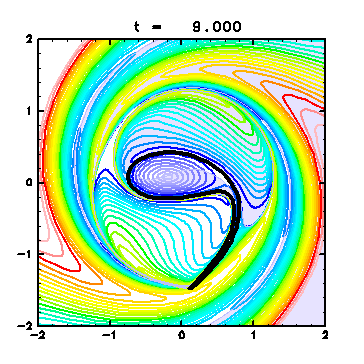# Deformation of a Vortex Patch and Fluid Mixing in Two-Dimensional Nondivergent Fluid

## -- Numerical Study with a Contour Dynamics Model --

Ryo Mizuta, Kenshi Horii and Shigeo Yoden ( Department of Geophysics, Kyoto University, Japan )

 Abstract:To examine vortex motions in two-dimensional nondivergent flows, a numerical study was done assuming idealized, simplified situations. Fundamental processes of deformation and mixing of the fluid are analyzed from several points of view. Assuming that vorticity is distributed piecewisely constant, time evolution of a vortex patch is calculated using a contour dynamics model. Assuming an unstable elliptical vortex as the initial state, the process of its deformation is examined; streamfunctions, finite-time Lyapunov exponents and so on are computed for understanding the deformation and mixing process of the vortex patch itself and the surrounding region. Streamfunctions in the co-rotating frame with the vortex show that the relative positioning of stagnation points to the vortex patch is an important element for the formation of a streamer. Finite-time Lyapunov exponents give a quantitative measure of local mixing.Text Appendix
 Received 25 January, 1998; in revised form 13 April, 1998